# Samacheer Kalvi 9th Maths Solutions Chapter 1 Set Language Ex 1.5

## Tamilnadu Samacheer Kalvi 9th Maths Solutions Chapter 1 Set Language Ex 1.5

Exercise 1.5 Class 9 Maths Samacheer Question 1.
Using the adjacent venn diagram, find the following sets:
(i) A – B
(ii) B – C
(iii) A’ ∪ B’
(vi) A’ ∩ B’
(v) (B ∪ C)’
(vi) A – (B ∪ C)
(vii) A – (B ∩ C)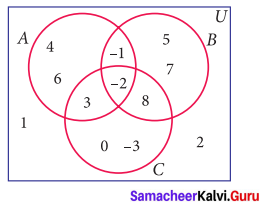Solution:
(i) A – B = {3, 4, 6}
(ii) B – C = {-1, 5, 7}
(iii) A’ ∪ B’
A’ = {1, 2, 0, -3, 5, 7, 8}
B’ = {-3, 0, 1, 2, 3, 4, 6)
A’ ∪ B’ = {-3, 0, 1, 2, 3, 4, 5, 6, 7, 8)

(iv) A’ ∩ B’
A’ ∩ B’ = {-3, 0, 1, 2}

(v) B ∪ C = {-3, -2, -1, 0, 3, 5, 7, 8}
(B ∪ C)’ = U – (B ∪ C)
= {-3, -2, -1, 0, 1,2, 3, 4, 5, 6, 7, 8} – {-3, -2, -1, 0, 3, 5, 7, 8}
(B ∪ C)’ = {1, 2, 4, 6}

(vi) A – (B ∪ C) = {-2, -1, 3, 4,6} – {-3, -2, -1, 0, 3, 5, 7, 8} = {4, 6}
A – (B ∩ C)
B ∩ C = {-2, 8}
A- (B ∩ C) = {-2, -1, 3, 4, 6} – {-2, 8} = {-1, 3, 4, 6}

9th Maths Exercise 1.5 Samacheer Kalvi Question 2.
If K = {a, b, d, e,f}, L = {b, c, d, g} and M {a, b, c, d, h} then find the following:
(i) K ∪ (L ∩ M)
(ii) K ∩ (L ∪ M)
(iii) (K ∪ L) ∩ (K ∪ M)
(iv) (K ∩ L) ∪ (K ∩ M) and verify distributive laws.
Solution:
K = {a, b, d, e, f}, L = {b, c, d, g} and M {a, b, c, d, h}
(i) K ∪ (L ∩ M)
L ∩ M = {b, c, d, g} ∩ {a, b, c, d, h} = {b, c, d}
K ∪ (L ∩ M) = {a, b, d, e, f } ∪ {b, c, d) = {a, b, c, d, e, f}

(ii) K ∩(L ∪ M)
L ∪ M = {a, b, c, d, g, h}
K ∩ (L ∪ M) = {a, b, d, e, f} ∩ {a, b, c, d, g, h} = {a, b, d}

(iii) (K ∪ L) ∩ (K ∪ M)
K ∪ L = {a, b, c, d, e, f, g}
K ∪ M = {a, b, c, d, e, f, h}
(K ∪ L) ∩ (K ∪ M) = {a, b, c, d, e,f}

(iv) (K ∩ L) ∪ (K ∩ M)
(K ∩ L) = {b, d)
(K ∩ M) = {a,b,d}
(K ∩ L) ∪ (K ∩ M) = {b, d} ∪ {a, b, d} = {a, b, d}

Distributive laws
K ∪ (L ∩ M) = (K ∪ L) ∩ (K ∪ M)
{a, b, c, d, e, f) = {a, b, c, d, e, f, g} ∩ {a, b, c, d, e, f, h}
= {a, b, c, d, e, f}
Thus Verified.
K ∩ (L ∪ M) = (K ∩ L) ∪ (K ∩ M)
{a, b, d} = {a, b, c, d, e, f, g} ∪ {a, b, c, d, e, f, h}
= {a, b, d}
Thus Verified.

9th Maths Exercise 1.5 Question 3.
If A = {x : x ∈ Z, -2 < x ≤ 4}, B = {x : x ∈ W, x ≤ 5}, C ={-4, -1, 0, 2, 3, 4}, then verify A ∪ (B ∩ C) = (A ∪ B) ∩ (A ∪ C).
Solution:
A = {x : x ∈ Z, -2 < x ≤ 4} = {-1, 0, 1, 2, 3, 4}
B = {x : x ∈ W, x ≤ 5} = {0, 1, 2, 3, 4, 5}
C = {-4, -1, 0, 2, 3, 4}
A ∪ (B ∩ C)
B ∩ C = {0, 1, 2, 3, 4, 5} ∩ {-4, -1, 0, 2, 3, 4} = {0, 2, 3, 4}
A ∪ (B ∩ C) = {-1, 0, 1, 2, 3, 4} ∪ (0, 2, 3, 4} ={-1, 0, 1, 2, 3, 4} …………. (1)
(A ∪ B) ∩ (A ∪ C)
A ∩ B = {0, 1, 2, 3, 4}
A ∩ C = {-1, 0, 2, 3, 4}
(A ∩ B) ∪ (A ∩ C) = {0, 1, 2, 3, 4} ∪ {-1, 0, 2, 3, 4}= {-1, 0, 1, 2, 3, 4} …………. (2)
From (1) and (2), it is verified that
A ∪ (B ∩ C) = (A ∪ B) ∩ (A ∪ C)

9th Maths Exercise 1.5 In Tamil Question 4.
Verify A ∪ (B ∩ C) = (A ∪ B) ∩ (A ∪ C) using Venn diagrams.
Solution:
L.H.S A ∪ (B ∩ C)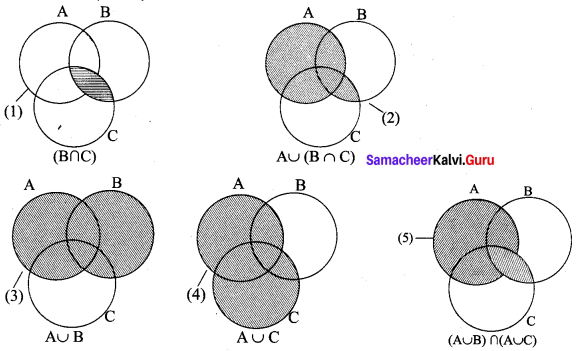From (2) and (5), it is verified that A ∪ (B ∩ C) = (A ∪ B) ∩ (A ∪ C)

9th Standard Maths Exercise 1.5 Question 5.
If A = {b, c, e, g, h}, B = {a, c, d, g, i} and C = {a, d, e, g, h}, then show that A – (B ∩ C) = (A – B) ∪ (A – C).
Solution:
A = {b, c, e, g, h}
B = {a, c, d, g, i}
C = {a, d, e, g, h}
B ∩ C = {a, d, g}
A – (B ∩ C) = {b, c, e, g, h} – {a, d, g} = {b, c, e, h} ……..… (1)
A- B = {b, c, e, g, h} – {a, c, d, g, i} = {b, e, h}
A – C = {b, c, e, g, h} – {a, d, e, g, h} = {b, c}
(A – B) ∪ (A – C) = {b, c, e, h} ………..… (2)
From (1) and (2) it is verified that
A – (B ∩ C) = (A – B) ∪ (A – C)

9th Maths Set Language Exercise 1.5 Question 6.
If A = {x : x = 6 n ∈ W and n < 6}, B = {x : x = 2n, n ∈ N and 2 < n ≤ 9} and C = {x : x = 3n, n ∈ N and 4 ≤ n < 10}, then show that A – (B ∩ C) = (A – B) ∪ (A – C)
Solution:
A = {x : x = 6n, n ∈ W, n < 6}
x = 6n
n = {0, 1, 2, 3, 4, 5}
⇒ x = 6 × 0 = 0
x = 6 × 1= 6
x = 6 × 2 = 12
x = 6 × 3 = 18
x = 6 × 4 = 24
x = 6 × 5 = 30
∴ A = {0, 6, 12, 18, 24, 30}

B = { x : x = 2n, n ∈ N, 2 < n ≤ 9}
n = {3, 4, 5, 6, 7, 8, 9}
x = 2 n
⇒ x = 2 × 3 = 6
2 × 4 = 8
2 × 5 = 10
2 × 6 = 12
2 × 7 = 14
2 × 8 = 16
2 × 9 = 18
∴ B {6, 8, 10, 12, 14, 16, 18}

C = { x : x = 3n, n ∈ N, 4 ≤ n < 10}
N = { 4, 5, 6, 7, 8, 9}
x = 3 × 4 = 12
⇒ x = 3 × 5 = 15
x = 3 × 6 = 18
x = 3 × 7 = 21
x = 3 × 8 = 24
x = 3 × 9 = 27
x = 2 × 9 = 18
∴ C = {12, 15, 18, 21, 24, 27}

A – (B ∩ C) = (A – B) ∪ (A – C)
L.H.S R.H.S
B ∩ C = {12,18}
A – (B ∩ C) = {0, 6, 12, 18, 24, 30} – {12, 18} = {0, 6, 24, 30} ……….…. (1)
(A – B) = {0, 24, 30}
(A – C) = {0, 6, 30}
(A – B) ∪ (A – C) = {0, 6, 24, 30} …………. (2)
From (1) and (2), it is verified that
A – (B ∩ C) = (A – B) ∪ (A – C).

Samacheer Kalvi Guru 9th Maths Question 7.
If A = {-2, 0, 1, 3, 5}, B = {-1, 0, 2, 5, 6} and C = {-1, 2, 5, 6, 7}, then show that A – (B ∪ C) = (A – B) ∩ (A – C).
Solution:
A = {-2, 0, 1, 3, 5},
B = {-1, 0, 2, 5, 6}
C ={-1, 2, 5, 6, 7}
B ∪ C = {-1, 0, 2, 5, 6, 7}
A – (B ∪ C) = {-2, 1, 3} …………. (1)
(A – B) = {-2, 1, 3}
(A – C) = {-2, 0, 1, 3}
(A – B) ∩ (A – C) = {-2, 1, 3} ………..… (2)
From (1) and (2), it is verified that . A – (B ∪ C) = (A – B) ∩ (A – C)

Kalvi Guru 9th Maths Question 8.
if A={y: y = $$\frac{a+1}{2}$$, a W and a 5},B = {y: y=$$\frac{2 n-1}{2}$$,n W and n < 5} and C={1,$$-\frac{1}{2}$$, 1, $$\frac{3}{2}$$, 2} then show that A – (B ∪ C) = (A – B) ∩ (A – C).
Solution: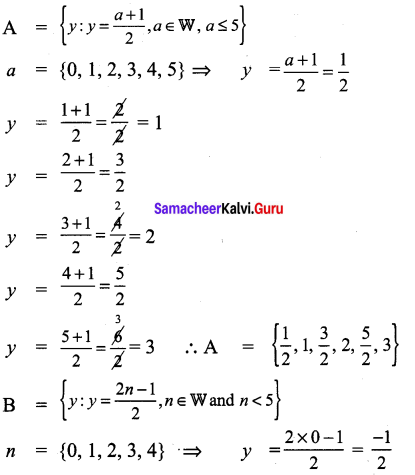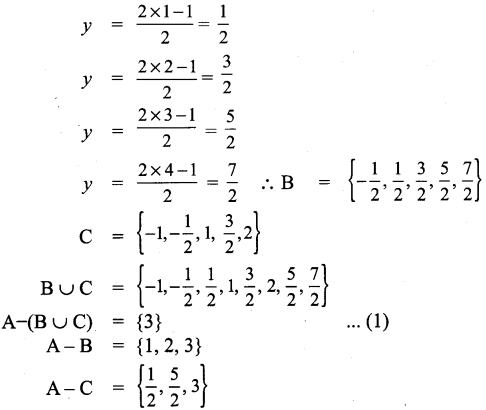(A – B) ∩ (A – C) = {3} …………. (2)
From (1) and (2), it is verified that A – (B ∪ C) = (A – B) ∩ (A – C).

9th Class Maths Exercise 1.5 Solution Question 9.
Verify A – (B ∩ C) = (A – B) ∪ (A – C) using Venn diagrams.
Solution: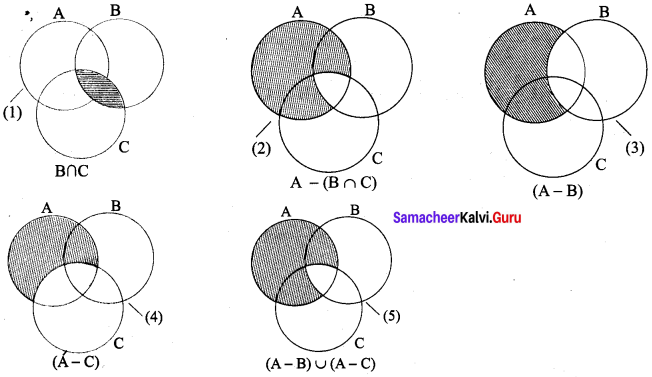∴ A – (B ∩ C) = (A – B) ∪ (A – C)
Hence it is proved.

Samacheer Kalvi Guru Maths 9th Question 10.
If U = {4, 7, 8, 10, 11, 12, 15, 16}, A = {7, 8, 11, 12} and B = {4, 8, 12, 15}, then verify De Morgan’s Laws for complementation.
U = {4, 7, 8, 10, 11, 12, 15, 16}
A = {7, 8, 11, 12}, B = {4, 8, 12, 15}
De Morgan’s Laws for complementation.
(A ∪ B)’ = A’ ∩ B’
A ∪ B = {4, 7, 8, 11, 12, 15}
(A ∪ B)’ = {4, 7, 8, 10, 11, 12, 15, 16} – {4, 7, 8, 11, 12, 15}
= {10, 16} ……………. (1)
A’ = {4, 10, 15, 16}
B’ = {7, 10, 11, 16}
A’ ∩ B’ = {10, 16} ………………(2)
From (1) and (2) it is verified that (A ∪ B)’ = A’ ∩ B’.

Samacheer Guru 9th Maths Question 11.
Verify (A ∩ B)’ = A’ ∪ B’ using Venn diagrams.
Solution:
(A ∩ B)’ = A’ ∪ B’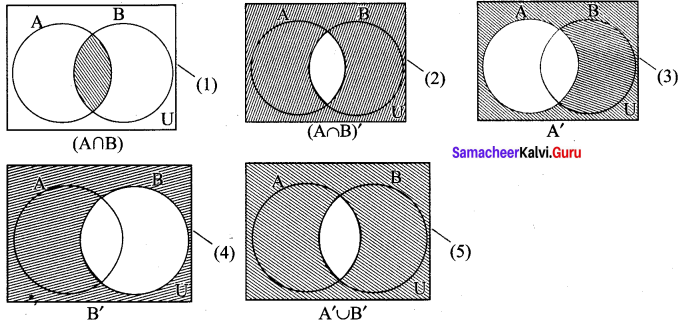(2) = (5)
∴ (A ∩ B)’ = A’ ∪ B’